Example Questions

Example Question #73 : Basic Squaring / Square Roots

Simplify: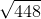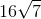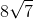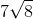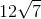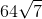Explanation:

To simplify square roots, we need to factor out perfect squares. In this case, it's.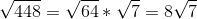Example Question #74 : Basic Squaring / Square Roots

Simplify: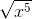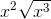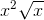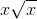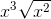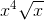Explanation:

To simplify the radical, we should factor out perfect squares.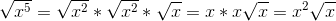Example Question #75 : Basic Squaring / Square Roots

Simplify: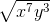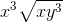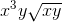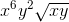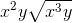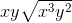Explanation:

To simplify the square roots, we need to factor out the perfect squares.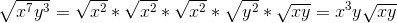Example Question #76 : Basic Squaring / Square Roots

Simplify: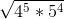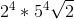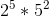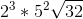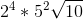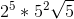Explanation:

To simplify the square roots, we need to factor out perfect squares.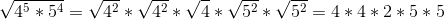We can combine to have two different bases. Remember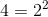.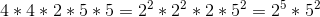Example Question #77 : Basic Squaring / Square Roots

Simplify: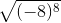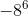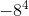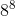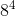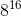Explanation:

To simplify the square root, we need to determine the value of the exponent and then simplify the radical.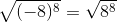Now let's find perfect squares.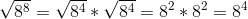Example Question #78 : Basic Squaring / Square Roots

Simplify: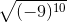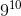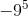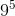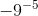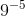Explanation:

To simplify the square root, we need to determine the value of the exponent and then simplify the radical.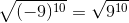Now let's find perfect squares.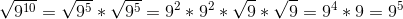Example Question #79 : Basic Squaring / Square Roots

Simplify: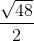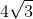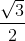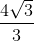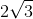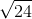Explanation:

To simplify the radical, we need to find perfect squares. Then if possible, we can reduc the fraction.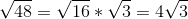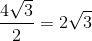Example Question #80 : Basic Squaring / Square Roots

Simplify: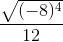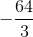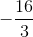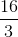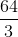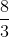Explanation:

To simplify the radical, let's deal with the parentheses first and apply the exponents. Reduce if necessary.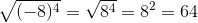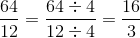Example Question #81 : Basic Squaring / Square Roots

Simplify: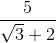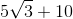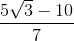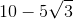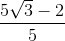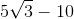Explanation:

To eliminate a radical expression, we need to multiply top and bottom by the conjugate which is opposite the sign in the expression. Then simplify if necessary.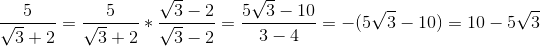Example Question #82 : Basic Squaring / Square Roots

Simplify: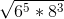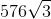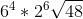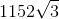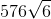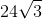Explanation:

To simplify square roots, we need to find perfect squares to factor out.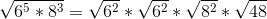We can also simplify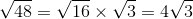Thus,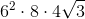We can compute the numbers outside to get a final answer of.